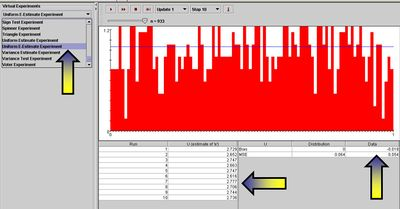# SOCR EduMaterials Activities Uniform E EstimateExperiment

## Description

This experiment is used to estimate the value of the natural number e using simulation. The e-estimate experiment allows us to generate a random sample $$X_1, X_2, ..., X_n$$ of size n from the uniform distribution on (0, 1). The distribution density is shown in blue in the graph, and on each update, the sample density is shown in red. On each update, the following statistic is recorded: U = minimum n for which the sum $$S = X_1+X_2+...+X_n > 1$$. That is, $$U= {\operatorname{argmin}}_n { \left (X_1+X_2+...+X_n > 1 \right )}$$, note that all $$X_i \ge 0$$, so such n exists.

## Goal

The purpose of the Uniform E-Estimate Experiment is to provide an interactive computer demonstration illustrating a simple idea behind a stochastic simulation for estimating the natural number e. If U = minimum n for which the sum $$S = X_1+X_2+...+X_n > 1$$, then the expected value of U, $$E(U)$$, is approximately equal to the natural number e~2.7182....

## Experiment

Go to the SOCR Experiments and select the Uniform E-Estimate Experiment from the drop-down list of experiments on the top left. The image below shows the initial view of this experiment:When pressing the play button, one trial will be executed and recorded in the distribution table below. The fast forward button symbolizes the n-th number of trials to be executed each time. The stop button ceases any activity and is helpful when the experimenter chooses “continuous run.” The fourth button will reset the entire experiment, deleting all previous information and data collected.

The “update” scroll indicates the number of trials (1, 10, 100, or 1,000) to be performed when selecting the fast forward button and the “stop” scroll indicates the maximum number of trials in the experiment.

Modifying the sample-size parameter (n) does not change the distribution graph of the events, but will significantly effect the estimates U when running the experiment.

## Applications

Estimation of the natural number e is very important in many science and technology developments and studies. There are deterministic algorithms as well as stochastic methods for estimating the value of e. Many of these provide up to 10-billion decimal place accuracy for e.

This experiment demonstrates an easy to understand, demonstrate and utilize protocol for a stochastic estimation of e. The algorithm may be significantly improved in terms of both speed of convergence and accuracy, relative to sample-size (n). However, the emphasis in this experiment is simplicity and simulation of a transcendental number in real-time using basic tools (sampling from uniform distribution).